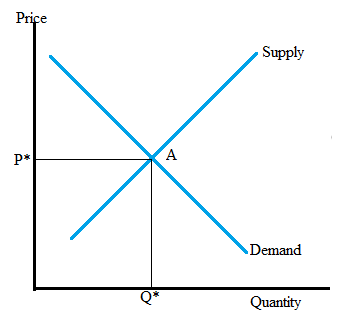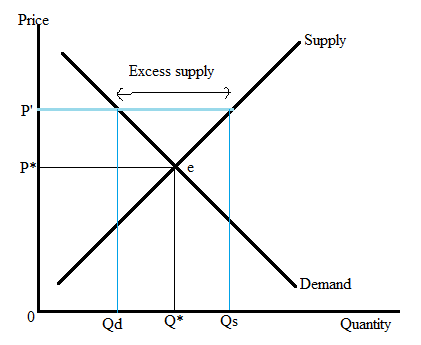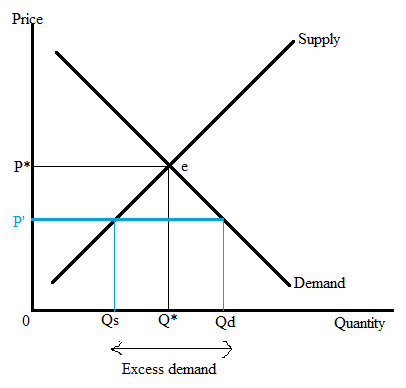# Define the concept of market equilibrium. Explain and demonstrate in a graph the process by which a market reaches equilibrium when there is a change in supply or demand (review the. Carefully explain how a shortage/ surplus gets eliminated through price adjustments following a change in supply or demand. Make specific reference to the law of demand and law of supply in your explanation.

Question
1 views
1. Define the concept of market equilibrium. Explain and demonstrate in a graph the process by which a market reaches equilibrium when there is a change in supply or demand (review the. Carefully explain how a shortage/ surplus gets eliminated through price adjustments following a change in supply or demand. Make specific reference to the law of demand and law of supply in your explanation.
check_circle

Step 1

The intersection point of supply and demand curves refers to the equilibrium point. At this point P* is the equilibrium price and Q* is the equilibrium quantity. At the equilibrium price, the consumer is willing to purchase Q* of quantity, which is equal to the quantity supplied by the producer. Because both the consumers and producers are content with this outcome and no one has the incentive to change their behavior.

In other words, at equilibrium, market demand is equal to the market supply. The equilibrium price and quantity will remain the same unless some other factors change the supply and demand.

The following graph represents the equilibrium situation in the market.Step 2

With the help of two scenarios, it can be seen that the point other than P and Q doesn’t meet the definition of equilibrium means represents the excess supply or demand in the market.

• The surplus in the market: If the price is above the equilibrium price that is, P’ > P.• In the above figure, P* and Q* are the equilibrium price and quantity respectively. If the new price is above the equilibrium price, then at this new price quantity supplied is greater than the quantity demanded. Because higher price attracts the producers for the shake of higher benefit and discourages the consumer due to the higher price. at a high price, the consumer is willing to buy less whereas the producer is willing to sell more. As a result, the situation of disequilibrium that is, excess supply generated in the market.
Step 3

2) Shortage in the market: If a price is below the equilibrium price that is, P’ > P.In the above figure, P* and Q* are the equilibrium price and quantity respectively. If the new price is below the equilibrium price, then at this new price quantity supplied is less than the quantity demanded. Because higher price discourages the producers, as their revenue fall and encourage the consumer due to lower price. At...

### Want to see the full answer?

See Solution

#### Want to see this answer and more?

Solutions are written by subject experts who are available 24/7. Questions are typically answered within 1 hour.*

See Solution
*Response times may vary by subject and question.
Tagged in